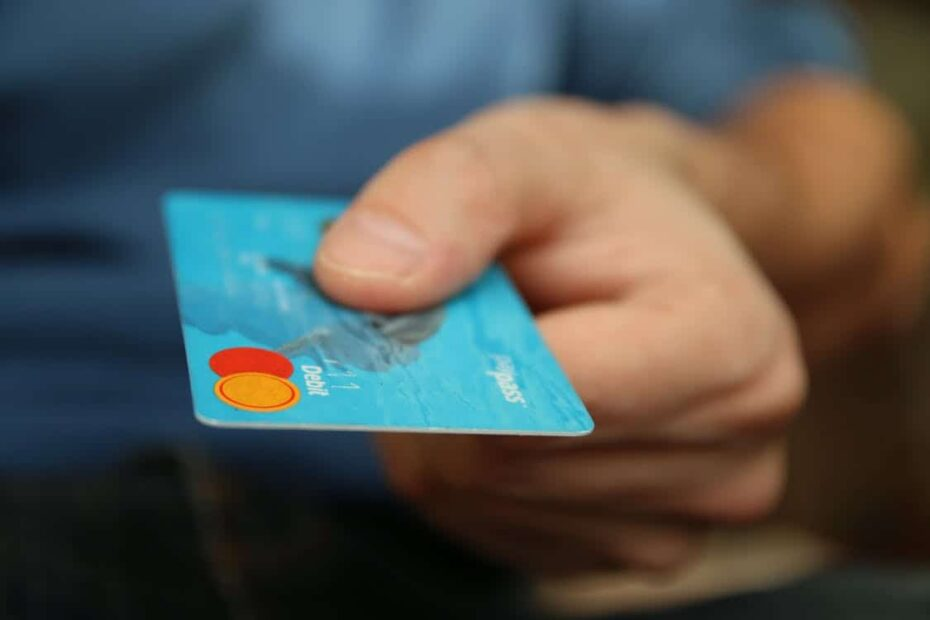Count Data Models and Consumer Purchasing Behavior AnalyticsWhen we talk about count data from an econometrics standpoint, we can understand that it is a form of data where the observations are in the form of non-negative integer values {0, 1, 2, 3, …}, and that these integers mostly come from the process of counting and not ranking. Also the focus of analysis in terms of marketing analytics, is commonly positioned on clarifying a dependent variable which is limited (also known as count data). The most common samples of customer behaviors are the number of specified products that have been procured or the number of customers that have visited any website within a one week time period.

Like many other customer analytics probability models, we can also use a certain type of distribution to understand the individual-level behaviors. A basic building block for count data is the Poisson distribution. A Poisson random variable (X) shows the number of occurrences of a certain event in either a space or time unit. Poisson distribution can be depicted in the equation shown below:(Eq.1)

Here, x = 0,1,2,… are non-negative integers that have no upper limit

Then, we assume a website has 10,000 registered users, and the users’ buying activities are logged. Moreover, to simplify the modeling even further, we can consider the conclusion of one user’s buying behavior in any one particular week.Figure 1

Thus, as per the Poisson model, we can include the equation and settings in the below equation.(Eq.2)

• We allow random variable Yi denote the number of times that an individual i made a purchase on the website in a certain time period unit.
• On an individual-level, Yi can be assumed to be the dispersed Poisson with mean λ i, (the average number of purchases in one single time period)

Now when it comes to practicing the science of marketing analytics, heterogeneity among various observations (buyers or consumers) is noted to be one of the most important issues that the model needs to deal with. Often, marketers mostly speak of the “80/20 rule”— which states that 80% of sales revenues come from just 20% of their customers. This pattern is also often observed: most consumers have just 1 or 2 buying activities, and a tiny percent of consumers also have huge amounts of activities. So we can see two types of heterogeneity that is, observed and unobserved. The Poisson Regression model takes care of the heterogeneity that is observed, and also the Negative Binomial Regression model deals with the second one.Figure 2 Density of Customers’ Purchases

• We assume that the exposure rates λ have a gamma distribution,so we can model the heterogeneity across the individuals

Based on the assumption that Table 1 shows many recordings of the number of times the customers purchased in one unit period along with their demographic features, such as level of education, number of children, etc.

 userid edu region householdsize age income child race Purchasing Times 6365661 5 1 2 11 7 0 1 1 6396922 2 2 2 8 4 0 1 1 8999933 4 3 5 10 3 1 1 1 9573834 . 4 2 10 5 1 1 2 9576277 . 1 3 8 7 1 1 5 9581009 . 2 2 7 5 1 1 1 9595310 4 2 2 8 2 1 1 6 9611445 2 4 2 11 6 1 1 2 9663372 4 4 3 9 7 1 1 28 9752844 3 4 2 7 3 1 1 2

Table 1 Sample Data of Customer Purchasing Activities

The Poisson regression model has been developed as the following through revised in the ordinary linear regression model

• An individual’s mean is related to her noticeable attributes through the function, guarantee λ>0(Eq.3)

• the link function like this, and after transformation

lnλ = lnλ0 + b1x1+b2x2 …….(Eq.4)

So we see that a Poisson Regression model is almost same as an ordinary linear regression, with mostly two variances. Firstly, the errors of regression follow a Poisson, and not normal distribution. Secondly, instead of modeling the dependent variable Y as a linear function of the independent variables, it starts modeling the natural log of the response variable, ln(Y), as a linear function of the determinants. The Poisson model makes an assumption that the mean and variance of errors are equal. However, usually when it comes to practice the variance of the errors is mostly bigger than the mean (although it can also be a bit lesser).

As many customer individualities are not noticed in practice, when we use the Poisson Regression model, we might miss some of the important information that causes the errors of omitted variables. In order to capture the unobserved heterogeneity among individuals, let λ0 (in Eq.3) vary across the individuals as per a Gamma distribution with parameters r and α,.So, we can get the following probability model, which is called the Negative Binomal Regression model.(Eq.5)

The negative binomial distribution is another type of the Poisson distribution in which the distribution’s parameter (λ0) is considered as a random variable itself. The variation of this parameter accounts for a variance of data that is greater than the mean, therefore the including an unobserved heterogeneity would improve the model’s good fit by a great extent.